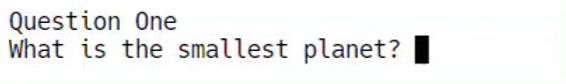top of page
top

## Python 5e - More Libraries## Clear Screen

Importing the os library and using the .system() command with the "clear" parameter will clear the screen.

The console won't clear on offline editors like IDLE but will work with many online editors like Replit.

import os

print("Hello")
os.
system("clear")
print("Bye")

Bye

Ask three trivia questions of your choice to the user and clear the screen between each one.

You should display the total they got correct after the third question - to do this you need to set a variable called correct to equal 0 at the start and then add 1 to correct each time a correct answer is given.

Example solution:## The Math Library

The math libraries contains several commands used for numbers:

• sqrt to find the square root of a number.

• ceil to round a decimal up to the nearest whole number and floor to round down to the nearest whole number.

• pi to generate the value of pi (π).

The sqrt command will find the square root of a number or variable placed in the brackets and return it as a decimal number.

from math import sqrt

8.0

The ceil command rounds a decimal up to the nearest integer and the floor command rounds a decimal down to the nearest integer.

from math import ceil, floor

Rounded up: 9

Rounded down: 8

The pi command generates a pi value accurate to 15 decimal places. Pi is used for many mathematical calculations involving circles.

The area of a circle is pi x radius².

The first example below uses 5.6 as the radius.

from math import pi

print("The area of the circle is" , area)

The area of the circle is 98.5203456165759

The example below uses an input to allow the user to enter a decimal (float) number for the radius. It also uses the ceil command to round the area up.

from math import pi, ceil

print("The area of the circle is" , ceil(area))

The area is 17

Clear Screen Task (Area of a Sphere)

The formula of a sphere is 4 x π x r² where π represents pi and r is the radius.

Use an input line to enter the radius and then calculate the area of the sphere.

Round the answer down to the nearest integer using floor and print it.

Example solution: